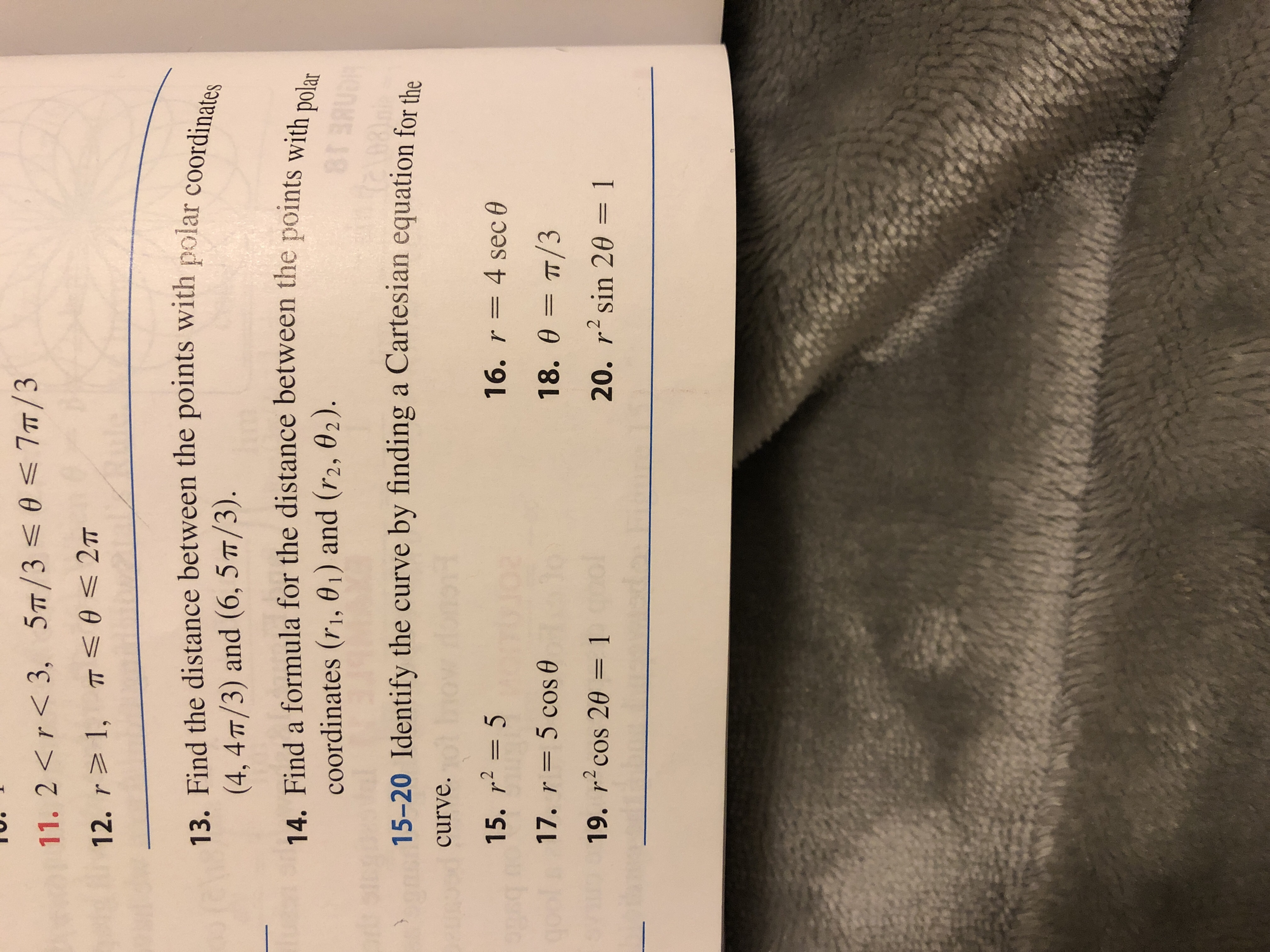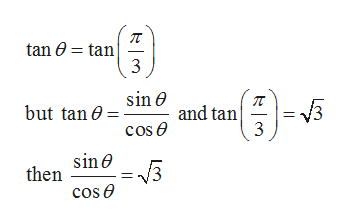# 11. 2 r< 3, 5T/30 7T/312. r 1, mT 2T13. Find the distance between the points with polar coordinates(4, 4TT/3) and (6, 5T/3).14. Find a formula for the distance between the points with polarcoordinates (r1, 01) and (r2, 02).8TBRUD15-20 Identify the curve by finding a Cartesian equation for thecurve.15. r2516. r4 sec 017. r 5 cos18. 0 TT/319. r2cos 20 = 120. r sin 20 1

Question
3 views

Identify the curve by finding a Cartesian equation for the curve.

18. θ = π/3help_outlineImage Transcriptionclose11. 2 r< 3, 5T/30 7T/3 12. r 1, mT 2T 13. Find the distance between the points with polar coordinates (4, 4TT/3) and (6, 5T/3). 14. Find a formula for the distance between the points with polar coordinates (r1, 01) and (r2, 02). 8TBRUD 15-20 Identify the curve by finding a Cartesian equation for the curve. 15. r25 16. r 4 sec 0 17. r 5 cos 18. 0 TT/3 19. r2cos 20 = 1 20. r sin 20 1 fullscreen
check_circle

Step 1

Given that

Step 2

To identify the curve by finding the Cartesian equation

Step 3

From the trigonome...help_outlineImage Transcriptionclosetan tan 3 sin e but tan0 and tan 3 cose sin e then cos e fullscreen

### Want to see the full answer?

See Solution

#### Want to see this answer and more?

Solutions are written by subject experts who are available 24/7. Questions are typically answered within 1 hour.*

See Solution
*Response times may vary by subject and question.
Tagged in
MathCalculus

### Other